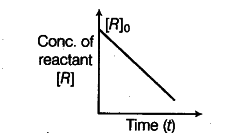# Predict the order of the reaction in this case

A reaction, reactant----------> product is represented
by (the graph)
(i) Predict the order of the reaction in this case.
(ii) What does the slope of the graph represent? Write the unit of the represented quantity.(i) The reaction is of zero order.
(ii) Slope of the graph = -k = d[R]/ dt
The unit of k, for zero order reaction is mol \${{L}^{-}}\$ \${{s}^{-}}\$